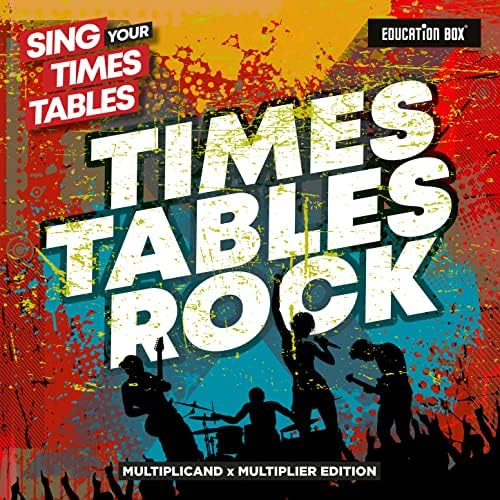Stream music and podcasts FREE on Amazon Music. No credit card required.# Sing Your Times Tables: Times Tables Rock (Multiplicand X Multiplier Edition)

Education Box & Sing Your Times Tables

22 SONGS • 49 MINUTES • MAY 07 2021

1
Two Times Table (Multiplicand X Multiplier Edition)
02:14
2
Three Times Table (Multiplicand x Multiplier Edition)
02:19
3
Four Times Table (Multiplicand x Multiplier Edition)
01:50
4
Five Times Table (Multiplicand x Multiplier Edition)
02:24
5
Six Times Table (Multiplicand x Multiplier Edition)
02:19
6
Seven Times Table (Multiplicand x Multiplier Edition)
02:23
7
Eight Times Table (Multiplicand x Multiplier Edition)
02:30
8
Nine Times Table (Multiplicand x Multiplier Edition)
02:16
9
Ten Times Table (Multiplicand x Multiplier Edition)
01:40
10
Eleven Times Table (Multiplicand x Multiplier Edition)
02:29
11
Twelve Times Table (Multiplicand x Multiplier Edition)
02:07
12
Two Times Table (No Answers) (Multiplicand x Multiplier Edition)
02:14
13
Three Times Table (No Answers) (Multiplicand x Multiplier Edition)
02:19
14
Four Times Table (No Answers) (Multiplicand x Multiplier Edition)
01:50
15
Five Times Table (No Answers) (Multiplicand x Multiplier Edition)
02:24
16
Six Times Table (No Answers) (Multiplicand x Multiplier Edition)
02:19
17
Seven Times Table (No Answers) (Multiplicand x Multiplier Edition)
02:23
18
Eight Times Table (No Answers) (Multiplicand x Multiplier Edition)
02:30
19
Nine Times Table (No Answers) (Multiplicand x Multiplier Edition)
02:16
20
Ten Times Table (No Answers) (Multiplicand x Multiplier Edition)
01:40
21
Eleven Times Table (No Answers) (Multiplicand x Multiplier Edition)
02:29
22
Twelve Times Table (No Answers) (Multiplicand x Multiplier Edition)
02:07
℗© 2021 Magic Star, a division of Sony Music Entertainment UK Limited